• ## Java流程控制语句

千次阅读 多人点赞 2019-09-28 23:05:26
流程控制语句就是用来控制程序中各语句执行的顺序。 分类 顺序结构 Java流程控制语句-顺序结构 分支结构（选择结构） Java流程控制语句-分支结构（选择结构） 循环结构 Java流程控制语句-循环结构 ...
定义
流程是指程序运行时，各语句的执行顺序。流程控制语句就是用来控制程序中各语句执行的顺序。
分类
顺序结构
Java流程控制语句-顺序结构
分支结构（选择结构）
Java流程控制语句-分支结构（选择结构）
循环结构
Java流程控制语句-循环结构


展开全文• ## Scala流程控制

千次阅读 2019-06-12 09:36:59
流程控制 在程序中，程序运行的流程控制决定程序是如何执行的。 流程控制说明 Scala 语言中控制结构和 Java 语言中控制结构基本相同，代码书写方式及理解方式都没什么太大的区别。 流程控制分值 顺序控制 分支控制 ...
流程控制
在程序中，程序运行的流程控制决定程序是如何执行的。
流程控制说明
Scala 语言中控制结构和 Java 语言中控制结构基本相同，代码书写方式及理解方式都没什么太大的区别。
流程控制分值

顺序控制
分支控制
循环控制

顺序控制说明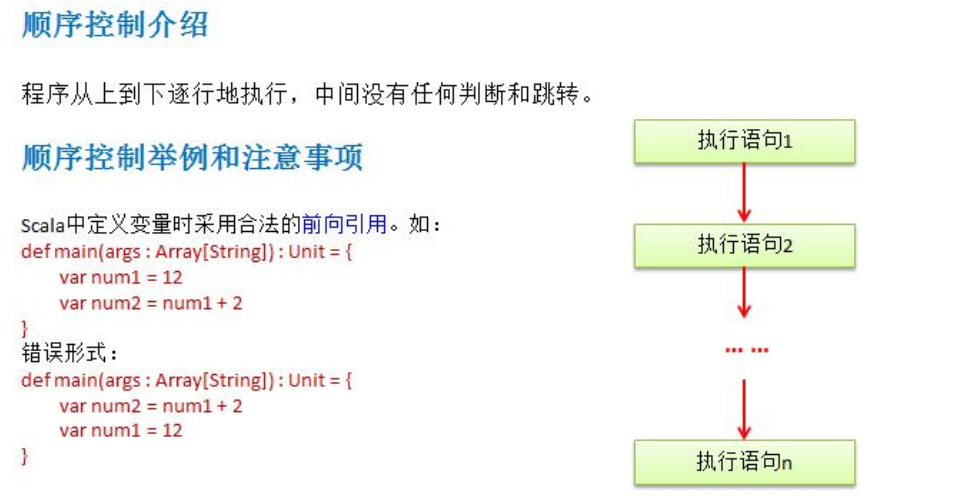分支控制
让程序有选择的执行，分支控制有三种

单分支
双分支
多分支

单分支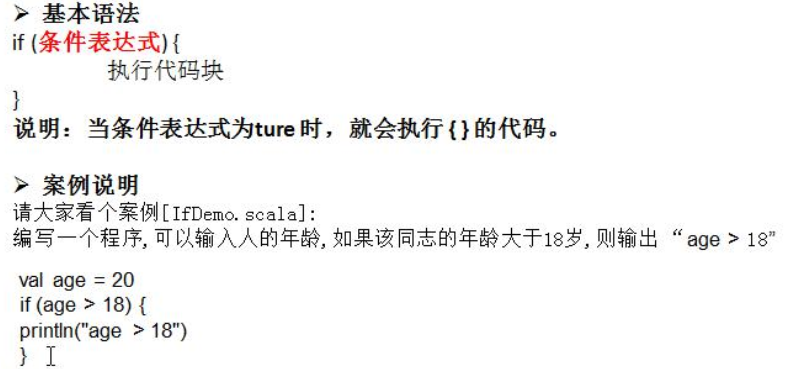import scala.io.StdIn

object Demo7 {

def main(args: Array[String]): Unit = {

println("您的年龄：")

if (age >= 18){
println("成年人")
}
}
}


双分支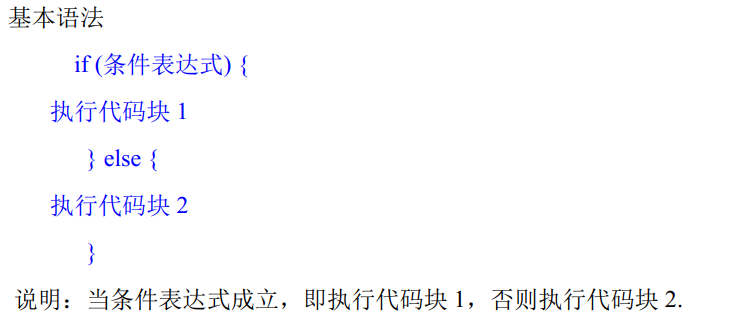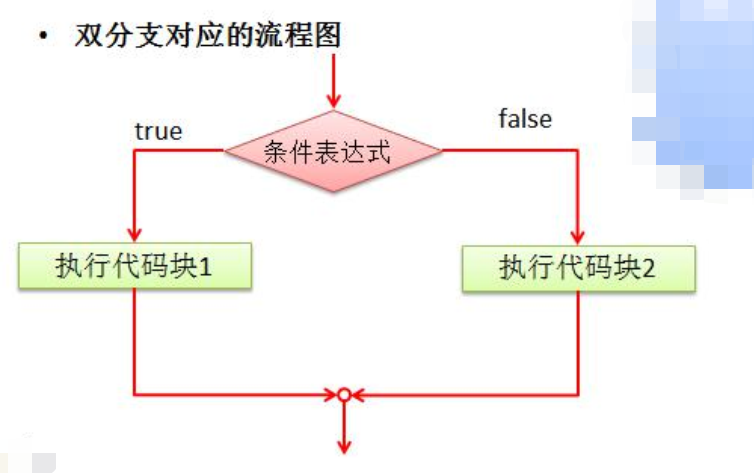import scala.io.StdIn

object Demo7 {

def main(args: Array[String]): Unit = {

println("您的年龄：")

if (age >= 18){
println("成年人")
} else {
println("未成年人")
}
}
}


多分支
if (条件表达式1){
执行代码块1
} else if (条件表达式2){
执行代码块2
}
......
else{
执行代码块n
}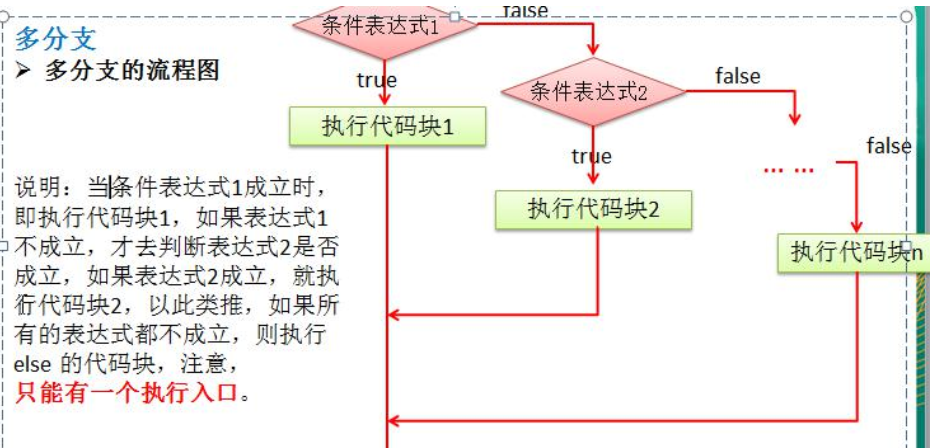import scala.io.StdIn

object Demo8 {
def main(args: Array[String]): Unit = {

println("输入您的分数：")

if (score == 100){
println("奖励30")
} else if (score >= 80) {
println("奖励10w")
} else if (score >= 60){
println("奖励2w")
} else {
println("没有奖励")
}
}
}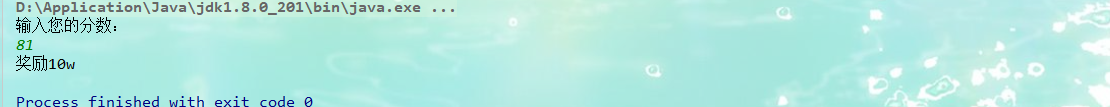展开全文scala
• java基础知识点02_流程控制 用户交互类Scanner： next()方法： 从有效字符开始，以空白作为结束符，所以不能得到带空格的字符串 public static void main(String[] args) { Scanner scanner = new Scanner(System...
java基础知识点02_流程控制

用户交互类Scanner：
next()方法：
从有效字符开始，以空白作为结束符，所以不能得到带空格的字符串

public static void main(String[] args) {
Scanner scanner = new Scanner(System.in);
System.out.println("输入：");
String str = scanner.next();
System.out.println(str);
scanner.close();
}

输入内容：Hello BLU
输出结果：Hello

nextLine()方法：
以Enter为结束符，可以得到含空格的字符串

public static void main(String[] args) {
Scanner scanner = new Scanner(System.in);
System.out.println("输入：");
String str = scanner.nextLine();
System.out.println(str);
scanner.close();
}

输入内容：Hello BLU
输出结果：Hello BLU

顺序结构：java的基本结构，语句一条条按顺序执行。
选择结构：
if单选择结构：
if(name.equals("BLU")){
System.out.println(name);
}

if双选择结构：
if(score>=60)){
System.out.println("及格");
}else {
System.out.println("不及格");
}

if多选择结构：
if(score==100)){
System.out.println("满分");
}else if(score>=90 && score<100) {
System.out.println("优秀");
}else if(score>=75 && score<90) {
System.out.println("良好");
}else if(score>=60 && score<75) {
System.out.println("及格");
}else if(score>=0 && score<60){
System.out.println("不及格");
}else {
System.out.println("不合法成绩");
}

嵌套if结构：
if(score>=0 && score<=100)){
if(score==100){
System.out.println("满分");
}else if(score>=90){
System.out.println("优秀");
}else if(score>=75){
System.out.println("良好");
}else if(score>=60){
System.out.println("及格");
}else {
System.out.println("不及格");
}
}else {
System.out.println("不合法成绩");
}

switch case 多选择结构：
switch(year){
case 2019:
System.out.println("去年");
break;
case 2020:
System.out.println("今年");
break;
case 2021:
System.out.println("明年");
default:
System.out.println("其他年份");
}

循环结构：
while循环输出1-100(先判断后执行)：

int i=0;
while(i<100){
i++;
System.out.println(i);
}

do..while循环输出1-100（先执行后判断，至少会执行一次）：

int i=0;
do{
i++;
System.out.println(i);
}while(i<=100);

for循环（让循环结构更简单,每次执行循环前先判断条件i<=100，执行一次循环后再i++）:
for(int i=1;i<=100;i++){
System.out.println(i);
}

增强for循环（主要用于数组和集合的遍历）：
int[] numbers = {1,2,3,4,5,6,7};
for(int x : numbers){
System.out.println(x);
}

break终止循环：
int i = 0;
while (i<=100){
i++;
if (i==30){
break;
}
System.out.println(i);
}

continue终止一次循环：
int i = 0;
while (i<=100){
i++;
if (i==30){
continue;
}
System.out.println(i);
}



展开全文java
• 运算符 程序流程控制
第三章 运算符

3.1 运算符介绍

运算符是一种特殊的符号，用以表示数据的运算、赋值和比较等。
1、算术运算符
2、赋值运算符
3、比较运算符(关系运算符)
4、逻辑运算符
5、位运算符

3.2 算术运算符

3.2.1 介绍

算术运算符(arithmetic)是对数值类型的变量进行运算的，在 Scala 程序中使用的非常多。

3.2.2 算术运算符一览图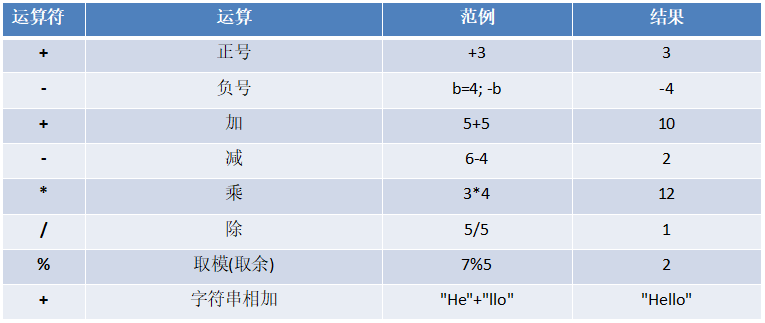3.2.3 案例演示

案例演示算术运算符的使用。

+, -, *, /, %   重点讲解 /、%
+, -, *         是一个道理，完全可以类推。
算数运算符的运算规则和 Java 一样。


示例代码如下：

package com.atguigu.chapter03.arithoper

object Demo01 {
def main(args: Array[String]): Unit = {
// / 的使用
var r1: Int = 10 / 3
println("r1=" + r1)   // 3
var r2: Double = 10 / 3
println("r2=" + r2)   // 3.0
var r3: Double = 10.0 / 3
println("r3=" + r3)   // 3.3333333333333335
println("r3=" + r3.formatted("%.2f")) // 3.33

// % 的使用
// 1、% 运算的原则：a % b = a - a/b * b
println(10 % 3)   // 1
println(-10 % 3)  // -1
println(-10 % -3) // -1
println(10 % -3)  // 1

// ++ 和 --
// 说明：在 Scala 中没有 ++ 和 -- 了，而是使用 +=1 和 -=1
var num1 = 10
// num1++ // error
// ++num1 // error
num1 += 1 // 替代了 num1++
num1 -= 1 // 替代了 num1--
}
}


3.2.4 细节说明

1、对于除号“/”，它的整数除和小数除是有区别的：整数之间做除法时，只保留整数部分而舍弃小数部分。例如：var x: Int = 10/3 结果是 3。
2、当对一个数取模时，可以等价 a%b=a-a/b*b ，这样我们可以看到取模的一个本质运算(和 java 的取模规则一样)。
3、注意：Scal中没有 ++、-- 操作符，需要通过 +=、-= 来实现同样的效果。

3.3.5 课堂练习

1、假如还有 97 天放假，问：xx 个星期零 xx 天。
2、定义一个变量保存华氏温度，华氏温度转换摄氏温度的公式为：5/9*(华氏温度-100)，请求出华氏温度对应的摄氏温度。[测试：232.5]
示例代码如下：

package com.atguigu.chapter03

object Exercise01 {
def main(args: Array[String]): Unit = {
// 1、假如还有 97 天放假，问：xx 个星期零 xx 天。
// (1) 搞清楚需求（读题）
// (2) 思路分析
// (3) 代码实现
val days = 97
printf("统计结果是：%d个星期零%d天", days / 7, days % 7)

println()

// 2、定义一个变量保存华氏温度，华氏温度转换摄氏温度的公式为：5/9*(华氏温度-100)，请求出华氏温度对应的摄氏温度。[测试：232.5]
val huashi = 232.5
val sheshi = 5 / 9.0 * (huashi - 100)
println("摄氏温度是" + sheshi.formatted("%.2f") + "℃")
}
}


输出结果如下：

统计结果是：13个星期零6天
摄氏温度是73.61℃


3.3 关系运算符(比较运算符)

3.3.1 基本介绍

1、关系运算符的结果都是 Boolean 型，也就是要么是 true，要么是 false。
2、关系表达式 经常用在 if结构的条件中或循环结构的条件中。
3、关系运算符的使用和 java 一样。

3.3.2 关系运算符一览图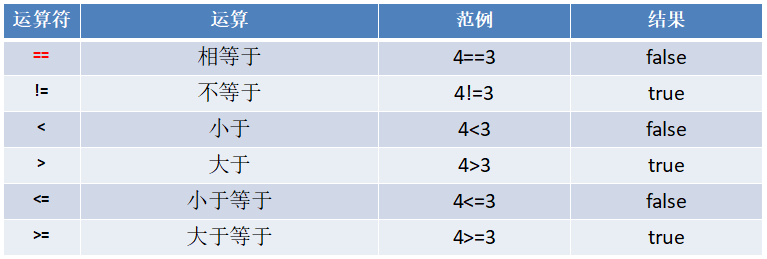3.3.3 案例演示

案例演示关系运算符的使用。
示例代码如下：

    var a = 9
var b = 8
println(a>b)  // true
println(a>=b) // true
println(a<=b) // false
println(a<b)  // false
println(a==b) // false
println(a!=b) // true
var flag: Boolean = a > b  // true


3.3.4 细节说明

1、关系运算符的结果都是 Boolean 型，也就是要么是 true，要么是 false。
2、关系运算符组成的表达式，我们称为关系表达式。【a > b】
3、比较运算符 "==" 不能误写成 "="。
4、使用陷阱: 如果两个浮点数进行比较，应当保证数据类型一致。

3.4 逻辑运算符

3.4.1 介绍

用于连接多个条件（一般来讲就是关系表达式），最终的结果也是一个 Boolean 值。

3.4.2 逻辑运算符一览图

假定变量 A 为 true，B 为 false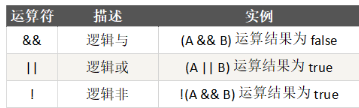示例代码如下：

    var a = true
var b = false
println("a && b = " + (a && b))  // false
println("a || b = " + (a || b))  // true
println("!(a && b) = " + !(a && b)) // true


3.5 赋值运算符

3.5.1 介绍

赋值运算符就是将某个运算后的值，赋给指定的变量。

3.5.2 赋值运算符的分类

1、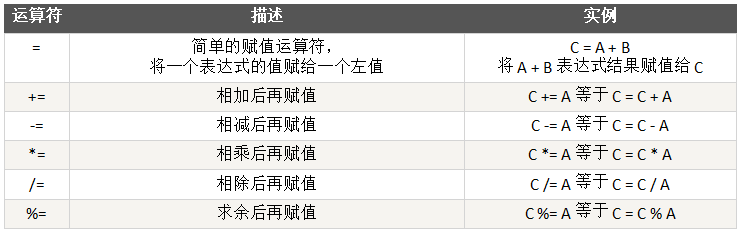2、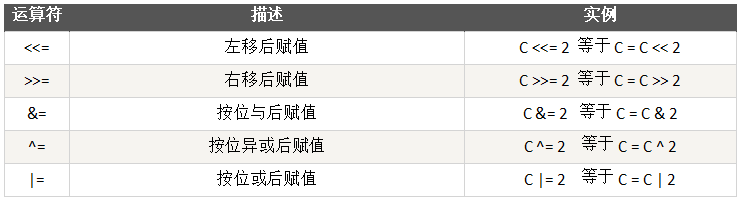说明：这部分的赋值运算涉及到二进制相关知识，其运行的规则和 Java 一样。
原码、反码、补码 详解：https://www.cnblogs.com/chenmingjun/p/8306863.html

3.5.3 案例演示

交换两个数的值。三种方法
示例代码如下：

    // 方式一：使用中间变量
var a = 10
var b = 99
println("a=" + a + "\tb=" + b)
// 交换
val t = a
a = b
b = t
println("a=" + a + "\tb=" + b)

// 方式二：使用加减运算方式
var a = 10
var b = 99
a = a + b
b = a - b  // => (a+b)-b = a = b
a = a - b  // => (a+b)-a = b = a

// 方式三：位运算方法：任意一个数与任意一个给定的值连续异或两次，值不变。
a = a ^ b;
b = a ^ b; // b = a ^ b = a ^ b ^ b = a
a = a ^ b; // a = a ^ b = a ^ a ^ b = b


3.5.4 赋值运算符特点

1、运算顺序从右往左。
2、赋值运算符的左边只能是变量，右边可以是变量、表达式、常量值/字面量。
3、复合赋值运算符等价于下面的效果：
比如：a += 3 等价于a = a + 3
示例代码如下：

package com.atguigu.chapter03.assignoper

object Demo01 {
def main(args: Array[String]): Unit = {
var num1 = 2
num1 <<= 2
println("num1=" + num1) // 8

var num2 = 2
num2 >>= 1
println("num2=" + num2) // 1

// 在 scala 中支持在代码块返回值
val res1 = {
90
}
println("res1=" + res1) // 90

val res2 = {
if (num1 > 1) "hello" else 100
}
println("res2=" + res2)
}
}


3.5.5 位运算符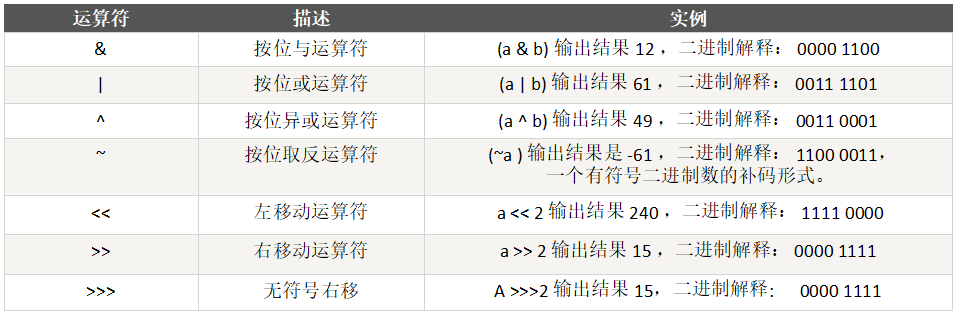说明: 位运算符的规则和Java一样

3.5.6 运算符的特别说明

Scala 不支持三目运算符，在 Scala 中使用 if – else 的方式实现。

val num = 5 > 4 ? 5 : 4  // 没有
val num = if (5 > 4) 5 else 4


变成设计思想：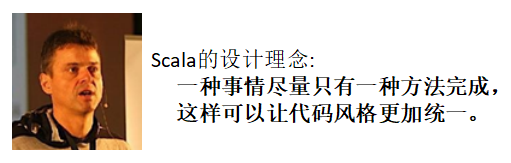3.5.7 练习

1、案例1：求两个数的最大值
2、案例2：求三个数的最大值
示例代码如下：

package com.atguigu.chapter03.notice

object Demo01 {
def main(args: Array[String]): Unit = {
// val num = 5 > 4 ? 5 : 4  // 没有
val num = if (5 > 4) 5 else 4

// 1、案例1：求两个数的最大值
// 2、案例2：求三个数的最大值
val n1 = 4
val n2 = 8
var res = if (n1 > n2) n1 else n2
println("res=" + res)

val n3 = 12
res = if (res > n3) res else n3
println("res=" + res)
}
}


输出结果如下：

res=8
res=12


3.6 运算符优先级

1、运算符有不同的优先级，所谓优先级就是表达式运算中的运算顺序。如下表，上一行运算符总优先于下一行。
2、只有单目运算符、赋值运算符是从右向左运算的。
3、运算符的优先级和 Java 一样。

运算符优先级一览图：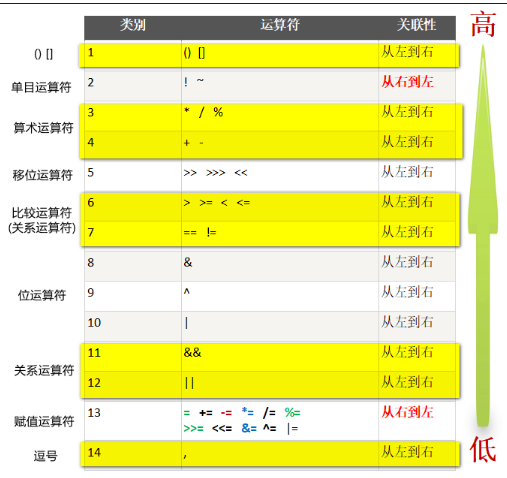运算符的优先级小结：
1、() []
2、单目运算符
3、算术运算符
4、移位运算符
5、比较运算符(关系运算符)
6、位运算符
7、关系运算符
8、赋值运算符
9、,逗号

3.7 键盘输入语句

3.7.1 介绍

在编程中，需要接收用户输入的数据，就可以使用键盘输入语句来获取。
Java 的实现步骤：
1、导入该类的所在包
2、创建该类对象（声明变量）
3、调用里面的功能

3.7.2 案例演示

要求：可以从控制台接收用户信息，【姓名，年龄，薪水】
1、回顾 Java 的实现
2、Scala的实现【import scala.io.StdIn】

示例代码如下：

package com.atguigu.chapter03.inputcon

import scala.io.StdIn

object Demo01 {
def main(args: Array[String]): Unit = {
// 要求：可以从控制台接收用户信息，【姓名，年龄，薪水】。
println("请输入姓名：")
println("请输入年龄：")
println("请输入薪水：")
printf("用户的信息为：name=%s age=%d sal=%.2f", name, age, sal)

println()

Cat.sayHi()
Cat.sayHello()
}
}

// 申明了一个对象（就是伴生对象）
object Cat extends AAA {
// 方法
def sayHi(): Unit = {
println("小猫喵喵叫")
}
}

trait AAA { // AAA 是特质，等价于 java 中的 interface + abstract class
// 方法
def sayHello(): Unit = {
println("hello")
}
}


输出结果如下：

请输入姓名：
chenmingjun
请输入年龄：
25
请输入薪水：
30000
用户的信息为：name=chenmingjun age=25 sal=30000.00
小猫喵喵叫
hello


第四章 程序流程控制

4.1 程序流程控制介绍

在程序中，程序运行的流程控制决定程序是如何执行的，是我们必须掌握的，主要有三大流程控制语句。
温馨提示：Scala 语言中控制结构和 Java 语言中的控制结构基本相同，在不考虑特殊应用场景的情况下，代码书写方式以及理解方式都没有太大的区别。
1、顺序控制
2、分支控制
3、循环控制

4.2 顺序控制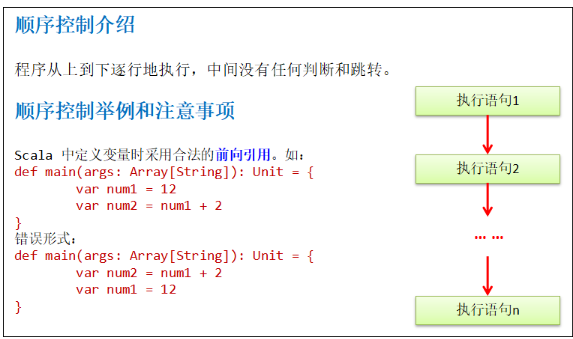4.3 分支控制 if-else

4.3.1 介绍

让程序有选择的的执行，分支控制有三种:
1、单分支
2、双分支
3、多分支

4.3.2 单分支的使用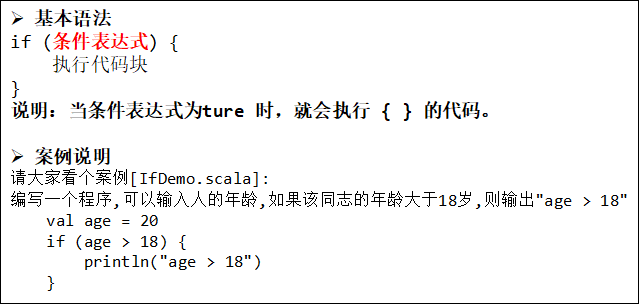示例代码如下：

package com.atguigu.chapter04.ifelse

// import scala.io.StdIn // 单独的引入一个StdIn
import scala.io._ // 下划线表示将 scala.io 包的所有内容一起引入

object IfDemo01 {
def main(args: Array[String]): Unit = {
println("请输入年龄：")
if (age > 18) {
println("age > 18")
}

// 小技巧：如何查看某个包下包含的内容
// 1、比如我们想看 scala.io 包下有什么内容
// 2、将光标放在 io 上即可，输入 Ctrl + B
// 2、将光标放在 StdIn 上即可，输入 Ctrl + B ，看的是 StdIn 的源码
scala.io.StdIn
}
}


4.3.3 双分支的使用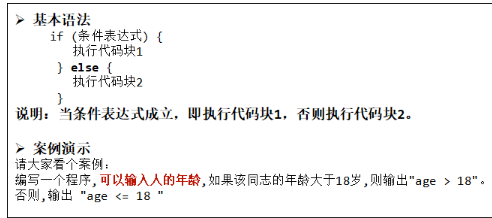小练习：判断一个年份是否是闰年，闰年的条件是符合下面二者之一：
(1) 年份能被4整除，但不能被100整除
(2) 年份能被400整除

4.3.4 多分支的使用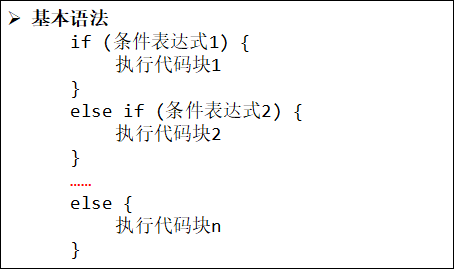多分支的流程图：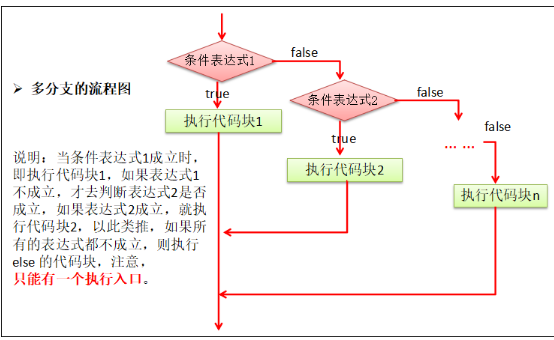案例演示：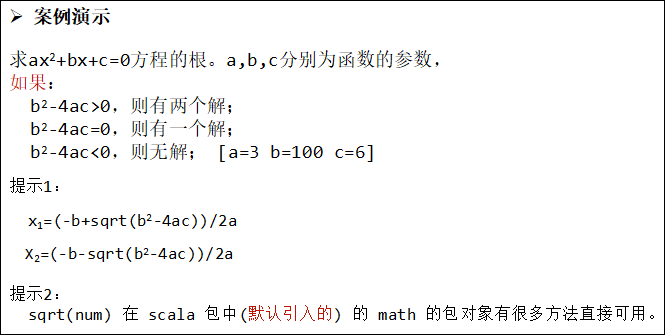示例代码如下：

package com.atguigu.chapter04.ifelse

import scala.math._ // 表示将 scala.math 的所有内容导入

/**
* 求ax2+bx+c=0方程的根。a,b,c分别为函数的参数，
* 如果：
* b2-4ac>0，则有两个解；
* b2-4ac=0，则有一个解；
* b2-4ac<0，则无解； [a=3 b=100 c=6]
* 提示1：
* x1=(-b+sqrt(b2-4ac))/2a
* X2=(-b-sqrt(b2-4ac))/2a
* 提示2：
* sqrt(num) 在 scala 包中(默认引入的) 的 math 的包对象有很多方法直接可用。
*/
object IfDemo02 {
def main(args: Array[String]): Unit = {
val a = 3
val b = 100
val c = 6
val m = b * b - 4 * a * c
var x1 = 0.0
var x2 = 0.0
if (m > 0) {
x1 = (-b + sqrt(m)) / 2 * a
x2 = (-b - sqrt(m)) / 2 * a
println("有两个解：x1=" + x1.formatted("%.2f") + " ，x2=" + x2.formatted("%.2f"))
} else if (m == 0) {
x1 = (-b + sqrt(m)) / 2 * a
println("有一个解：x =" + x1.formatted("%.2f"))
} else {
println("无解")
}
}
}


输出结果如下：

有两个解：x1=-0.54 ，x2=-299.46


4.3.5 注意事项

分支控制 if-else 注意事项
1、如果大括号{}内的逻辑代码只有一行，大括号可以省略，这点和 java 的规定一样。
2、Scala 中任意表达式都是有返回值的，也就意味着 if else 表达式其实是有返回结果的，具体返回结果的值取决于执行满足条件的代码体的最后一行内容。
示例代码如下：

    // Scala 中任意表达式都是有返回值的，也就意味着 if else 表达式其实是有返回结果的，具体返回结果的值取决于执行满足条件的代码体的最后一行内容。
val age = 70
val result = if (age > 20) {
println("hello age > 20")
9 + 10
"yes ok"
} else {
7
}
println("result=" + result) // yes ok


3、Scala 中是没有三元运算符，因为可以这样简写。
示例代码如下：

    // Java
int result = flag ? 1 : 0
// Scala
val result = if (flag) 1 else 0   // 因为 scala 的 if-else 是有返回值的，因此，本身这个语言也不需要三元运算符了，并且可以写在同一行，类似三元运算！


4、小案例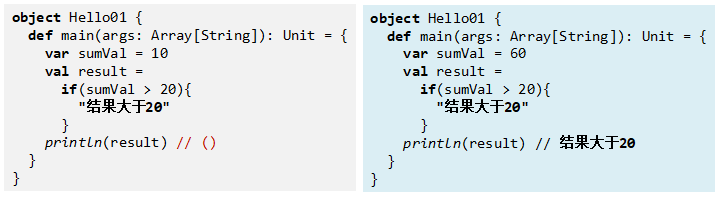4.4 嵌套分支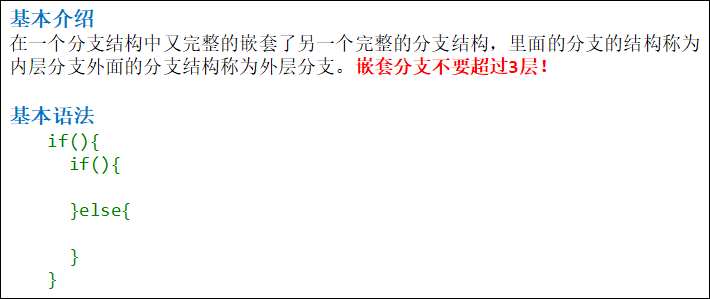应用案例1
参加百米运动会，如果用时8秒以内进入决赛，否则提示淘汰。并且根据性别提示进入男子组或女子组。
输入成绩和性别，进行判断。
示例代码如下：

package com.atguigu.chapter04.ifelse

import scala.io.StdIn

/**
* 参加百米运动会，如果用时8秒以内进入决赛，否则提示淘汰。并且根据性别提示进入男子组或女子组。
* 输入成绩和性别，进行判断。
*/
object Exercise02 {
def main(args: Array[String]): Unit = {
println("请输入成绩：")
if (speed <= 8) {
println("请输入性别：")
if (gender == '男') {
println("进入男子组")
} else {
println("进入女子组")
}
} else {
println("你被淘汰了")
}
}
}


应用案例2
出票系统：根据淡旺季的月份和年龄，打印票价。
4_10 旺季：
成人（18-60）：60
儿童（ < 18）：半价   老人（ > 60）：1/3
淡季：
成人：40
其他：20
示例代码如下：

package com.atguigu.chapter04.ifelse

import scala.io.StdIn

/**
* 出票系统：根据淡旺季的月份和年龄，打印票价。
* 4_10 旺季：
* 成人（18-60）：60
* 儿童（ < 18）：半价
* 老人（ > 60）：1/3
* 淡季：
* 成人：40
* 其他：20
*/
object Exercise03 {
def main(args: Array[String]): Unit = {
val ticket = 60
println("请输入月份：")
if (month <= 10 && month >= 4) {
println("请输入年龄：")
if (age < 18) {
println("你的票价是" + ticket / 2 + "元")
} else if (age > 60) {
println("你的票价是" + ticket / 3 + "元")
} else {
println("你的票价是" + ticket + "元")
}
} else {
println("请输入年龄：")
if (age >= 18 && age <= 60) {
println("你的票价是" + ticket / 3 * 2 + "元")
} else {
println("你的票价是" + ticket / 3 + "元")
}
}
}
}


4.5 switch 分支结构

在 scala 中没有 switch，而是使用模式匹配来处理。
模式匹配涉及到的知识点较为综合，因此我们放在后面讲解。【match-case】

4.6 for 循环控制

4.6.1 基本介绍

Scala 也为 for 循环这一常见的控制结构提供了非常多的特性，这些 for 循环的特性被称为 for 推导式（for comprehension）或 for 表达式（for expression）。

4.6.2 范围数据循环方式1

基本案例：

for (i <- 1 to 3) {
println(i)
}


说明：
i 表示循环的变量，<- 规定， to 规定
i 将会从 1-3 循环，前后闭合

输出10句 "hello, world!"
示例代码如下：

package com.atguigu.chapter04.myfor

object ForToDemo {
def main(args: Array[String]): Unit = {
// 输出10句 "hello, world!"
val start = 1
val end = 10
// 说明
// 1. start 从哪个数开始循环
// 2. to 是关键字
// 3. end 循环结束的值
// 4. start to end 表示【前后闭合】
for (i <- start to end) {
println("hello, world!" + i)
}

println("---------")

// 说明：for 推导式，也可以直接对集合进行遍历
var list = List("hello", 10, 30, "tom")
for (item <- list) {
println("item=" + item)
}
}
}


4.6.3 范围数据循环方式2

基本案例：

for(i <- 1 until 3) {
println(i)
}


说明：
这种方式和前面的区别在于 i 是从 1 到 3-1=2
前闭合后开的范围，和 java 的 arr.length() 类似
for (int i = 0; i < arr.lenght; i++){ }

输出10句 "hello, world!"
示例代码如下：

package com.atguigu.chapter04.myfor

object ForUntilDemo {
def main(args: Array[String]): Unit = {
// 输出10句 "hello, world!"
val start = 1
val end = 11
// 说明
// 1. start 从哪个数开始循环
// 2. until 是关键字
// 3. end 循环结束的值
// 4. start to until 表示【前闭合后开】
for (i <- start until end) {
println("hello, world!" + i)
}
}
}


4.6.4 循环守卫

基本案例：

for(i <- 1 to 3 if i != 2) {
println(i)
}


基本案例说明：
循环守卫，即循环保护式（也称条件判断式：守卫）。保护式为 true了，则进入循环体内部，为 false 则跳过，类似于 continue。
上面的代码等价：

for (i <- 1 to 3) {
if (i != 2) {
println(i)
}
}


示例代码如下：

package com.atguigu.chapter04.myfor

object ForGuardDemo {
def main(args: Array[String]): Unit = {
for(i <- 1 to 3 if i != 2) {
println(i)
}

println("---------")

// 上面代码等价于
for (i <- 1 to 3) {
if (i != 2) {
println(i)
}
}
}
}


输出结果如下：

1
3
1
3


4.6.5 引入变量

基本案例：

for(i <- 1 to 3; j = 4 - i) {
println(j)
}


对基本案例说明：
没有关键字，所以范围后一定要加 分号 来隔断逻辑。
上面的代码等价：

for ( i <- 1 to 3) {
val j = 4 - i
println(j)
}


示例代码如下：

package com.atguigu.chapter04.myfor

object ForVarDemo {
def main(args: Array[String]): Unit = {
for(i <- 1 to 3; j = 4 - i) {
println(j)
}

println("---------")

// 上面代码等价于
for ( i <- 1 to 3) {
val j = 4 - i
println(j)
}
}
}


输出结果如下：

3
2
1
3
2
1


4.6.6 嵌套循环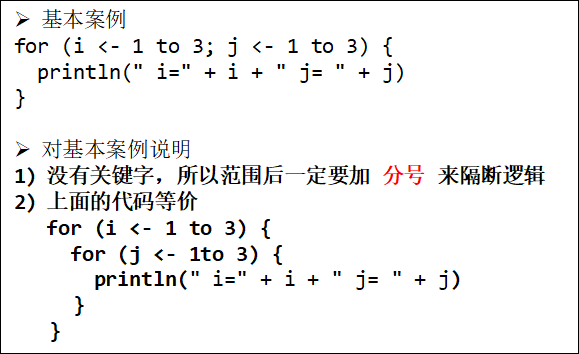示例代码如下：

package com.atguigu.chapter04.myfor

object ForMultiDemo {
def main(args: Array[String]): Unit = {
for (i <- 1 to 3; j <- 1 to 3) {
println(" i=" + i + " j= " + j)
}

println("---------")

// 上面代码等价于
for (i <- 1 to 3) {
for (j <- 1 to 3) {
println(" i=" + i + " j= " + j)
}
}
}
}


输出结果如下：

 i=1 j= 1
i=1 j= 2
i=1 j= 3
i=2 j= 1
i=2 j= 2
i=2 j= 3
i=3 j= 1
i=3 j= 2
i=3 j= 3
---------
i=1 j= 1
i=1 j= 2
i=1 j= 3
i=2 j= 1
i=2 j= 2
i=2 j= 3
i=3 j= 1
i=3 j= 2
i=3 j= 3


4.6.7 循环返回值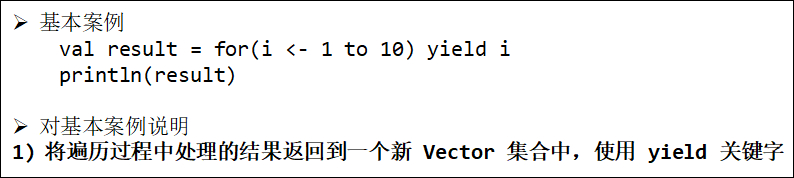示例代码如下：

package com.atguigu.chapter04.myfor

object ForYieldDemo {
def main(args: Array[String]): Unit = {
// 说明 val result = for(i <- 1 to 10) yield i 含义
// 1. 对 1 to 10 进行遍历
// 2. yield i 将每次循环得到 i 放入到集合新的集合 Vector 中，并返回给 result
// 3. i 这里可以是一个代码块，这就意味我们可以对 i 进行处理进行处理，并返回给新的集合
val result = for (i <- 1 to 10) yield i
println(result)

// 4. 下面的这个方式，就体现出 scala 一个重要的语法特点：就是将一个集合中个各个数据进行处理，并返回给新的集合
val result1 = for (i <- 1 to 10) yield {
if (i % 2 == 0) {
i + "是偶数"
} else {
i + "不是偶数"
}
}
println(result1)
}
}


输出结果如下：

Vector(1, 2, 3, 4, 5, 6, 7, 8, 9, 10)
Vector(1不是偶数, 2是偶数, 3不是偶数, 4是偶数, 5不是偶数, 6是偶数, 7不是偶数, 8是偶数, 9不是偶数, 10是偶数)


4.6.8 使用花括号{}代替小括号()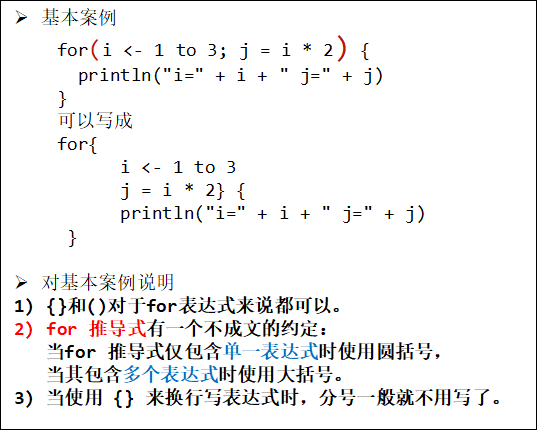4.6.9 注意事项和细节说明

1、scala 的 for 循环形式和 java 是较大差异，这点请同学们注意，但是基本的原理还是一样的。
2、scala 的 for 循环的步长如何控制？【for (i <- Range(1, 3, 2)】
3、思考题：如何使用循环守卫控制步长？
示例代码如下：

package com.atguigu.chapter04.myfor

object ForStep {
def main(args: Array[String]): Unit = {
for (i <- 1 to 10) {
println("i=" + i)
}
// 步长控制为2
println("----------")
// Range(1, 10, 2)的对应的构建方法如下：
// def apply(start: Int, end: Int, step: Int): Range = new Range(start, end, step)
for (i <- Range(1, 10, 2)) {
println("i=" + i)
}

// 控制步长的第二种方式：for循环守卫
println("----------")
for (i <- 1 to 10 if i % 2 == 1) {
println("i=" + i)
}
}
}


输出结果如下：

i=1
i=2
i=3
i=4
i=5
i=6
i=7
i=8
i=9
i=10
----------
i=1
i=3
i=5
i=7
i=9
----------
i=1
i=3
i=5
i=7
i=9


4.6.10 for 循环练习题

1、打印 1~100 之间所有是 9 的倍数的整数的个数及总和。
2、完成下面的表达式输出
示例代码如下：

package com.atguigu.chapter04.myfor

object ForExercise01 {
def main(args: Array[String]): Unit = {
// 1、打印1~100之间所有是9的倍数的整数的个数及总和
var count = 0
var sum = 0
val start = 1
val end = 100
for (i <- 1 to 100) {
if (i % 9 == 0) {
count += 1
sum += i
}
}
println("个数是：" + count + "，总和是：" + sum)
printf("count=%d, sum=%d", count, sum)

// 2、完成下面的表达式输出
val num = 6
for (i <- 0 to num) {
printf("%d + %d = %d\n", i, (num - i), num)
}
}
}


输出结果如下：

个数是：11，总和是：594
count=11, sum=5940 + 6 = 6
1 + 5 = 6
2 + 4 = 6
3 + 3 = 6
4 + 2 = 6
5 + 1 = 6
6 + 0 = 6


4.7 while 循环控制

4.7.1 基本语法

基本语法与 Java 相同

循环变量初始化
while (循环条件) {
循环体(语句)
循环变量迭代
}

4.7.2 while 循环应用实例

1、画出流程图
2、输出10句 "hello world"。
示例代码如下：

package com.atguigu.chapter04.mywhile

object WhileDemo01 {
def main(args: Array[String]): Unit = {
// 输出10句 "hello world"。
var i = 0
while (i < 10) {
println("hello world" + i)
i += 1
}
}
}


4.7.3 注意事项和细节说明

1、循环条件是返回一个布尔值的表达式。
2、while 循环是先判断再执行语句。
3、与 if 语句不同，while 语句本身没有值，即整个 while 语句的结果是 Unit 类型的()。
4、因为 while 中没有返回值，所以当要用该语句来计算并返回结果时，就不可避免的使用变量，而变量需要声明在 while 循环的外部，那么就等同于循环的内部对外部的变量造成了影响，所以不推荐使用 while，而是推荐使用 for 循环。(注意：不是禁止使用！)
5、Scala 中任意表达式都是有返回值的，也就意味着 if else 表达式其实是有返回结果的，具体返回结果的值取决于执行满足条件的代码体的最后一行内容。
纯函数：Scala 设计者非常提倡使用函数的递归来解决问题，函数自己调用自己，函数的变量在函数的内部变化，而不会对外部的变量造成了影响。即纯函数概念。

4.8 do…while 循环控制

4.8.1 基本语法

循环变量初始化;
do {
循环体(语句)
循环变量迭代
} while (循环条件)

4.8.2 do…while 循环应用实例

1、输出10句 "hello world"。
2、计算1—100的和。
3、统计1-200之间能被5整除但不能被3整除的个数。
示例代码如下：

package com.atguigu.chapter04.mydowhile

object DoWhileDemo01 {
def main(args: Array[String]): Unit = {
// 1、输出10句 "hello world"。
var i = 0
do {
println("hello wprld" + i)
i += 1
} while (i < 10)

// 2、计算1—100的和。
var j = 0
var sum = 0
do {
sum += j
j += 1
} while (j <= 100)
println("sum=" + sum)

// 3、统计1-200之间能被5整除但不能被3整除的个数。
var k = 1
var count = 0
do {
if (k % 5 == 0 && k % 3 != 0) {
count += 1
}
k += 1
} while (k <= 200)
println("count=" + count)
}
}


输出结果如下：

hello wprld0
hello wprld1
hello wprld2
hello wprld3
hello wprld4
hello wprld5
hello wprld6
hello wprld7
hello wprld8
hello wprld9
sum=5050
count=27


4.8.3 注意事项和细节说明

1、循环条件是返回一个布尔值的表达式。
2、do…while 循环是先执行，再判断。
3、和 while 一样，因为 do…while 中没有返回值，所以当要用该语句来计算并返回结果时，就不可避免的使用变量，而变量需要声明在 do…while 循环的外部，那么就等同于循环的内部对外部的变量造成了影响，所以不推荐使用，而是推荐使用 for 循环。

4.9 多重循环控制

4.9.1 介绍

1、将一个循环放在另一个循环体内，就形成了嵌套循环。其中 forwhile, do…while 均可以作为外层循环和内层循环。【建议一般使用两层，最多不要超过3层】
2、实质上，嵌套循环就是把内层循环当成外层循环的循环体。当只有内层循环的循环条件为 false 时，才会完全跳出内层循环，才可结束外层的当次循环，开始下一次的循环。
3、设外层循环次数为 m 次，内层为 n 次， 则内层循环体实际上需要执行 m*n=mn 次。

4.9.2 应用实例

1、统计三个班成绩情况，每个班有 5 名同学，求出各个班的平均分和所有班级的平均分[学生的成绩从键盘输入]。
2、统计三个班及格人数，每个班有 5 名同学。
示例代码如下：

package com.atguigu.chapter04.mutlifor

import scala.io.StdIn

/**
* 2、统计三个班及格人数，每个班有 5 名同学。
*/
object Exercise02 {
def main(args: Array[String]): Unit = {
val classNum = 3
val stuSum = 5

var score = 0.0
var classScoreSum = 0.0
var totalScore = 0.0

var count = 0

for (i <- 1 to classNum) {
// 清零操作
classScoreSum = 0.0
for (j <- 1 to stuSum) {
printf("请输入第%d班级的第%d个学生的成绩：\n", i, j)
if (score >= 60) {
count += 1
}
classScoreSum += score
}
printf("第%d班级的平均分为：%.2f\n", i, classScoreSum / stuSum)
totalScore += classScoreSum
}
printf("所有班级的平均分为：%.2f\n", totalScore / classNum)
printf("所有班级的及格人数为：%d\n", count)
}
}


3、打印出九九乘法表。
示例代码如下：

package com.atguigu.chapter04.mutlifor

/**
* 3、打印出九九乘法表。
*/
object Exercise03 {
def main(args: Array[String]): Unit = {
val num = 9
for (i <- 1 to num) { // 行数
for (j <- 1 to i) { // 列数=行数
printf("%d * %d = %d\t", j, i, i * j)
}
println()
}
}
}


输出结果如下：

1 * 1 = 1
1 * 2 = 2    2 * 2 = 4
1 * 3 = 3    2 * 3 = 6   3 * 3 = 9
1 * 4 = 4    2 * 4 = 8   3 * 4 = 12  4 * 4 = 16
1 * 5 = 5    2 * 5 = 10  3 * 5 = 15  4 * 5 = 20  5 * 5 = 25
1 * 6 = 6    2 * 6 = 12  3 * 6 = 18  4 * 6 = 24  5 * 6 = 30  6 * 6 = 36
1 * 7 = 7    2 * 7 = 14  3 * 7 = 21  4 * 7 = 28  5 * 7 = 35  6 * 7 = 42  7 * 7 = 49
1 * 8 = 8    2 * 8 = 16  3 * 8 = 24  4 * 8 = 32  5 * 8 = 40  6 * 8 = 48  7 * 8 = 56  8 * 8 = 64
1 * 9 = 9    2 * 9 = 18  3 * 9 = 27  4 * 9 = 36  5 * 9 = 45  6 * 9 = 54  7 * 9 = 63  8 * 9 = 72  9 * 9 = 81


4.10 while 循环的中断

4.10.1 基本说明

Scala 内置控制结构特地去掉了 break 和 continue，是为了更好的适应函数化编程，推荐使用函数式的风格解决 break 和 contine 的功能，而不是一个关键字。

4.10.2 break 的应用实例

代码说明：(看源码分析 重要)
示例代码如下：

package com.atguigu.chapter04.mybreak

import util.control.Breaks._

object WhileBreakDemo {
def main(args: Array[String]): Unit = {

var n = 1
// breakable() 函数
// 说明
// 1、breakable() 函数是一个高阶函数：可以接收函数的函数就是高阶函数。
/*
def breakable(op: => Unit) {
try {
op
} catch {
case ex: BreakControl =>
if (ex ne breakException) throw ex
}
}
*/
// (1) op: => Unit 表示接收的参数是一个没有输入，也没有返回值的函数，即可以简单地理解可以接收一段代码块。
// (2) breakable() 对 break() 抛出的异常做了处理，这样剩余的代码就可以继续执行了。
// (3) 当我们传入的是代码块时，scala 程序员一般会将 breakable() 的 () 改为 {}
breakable {
while (n <= 20) {
n += 1
println("n=" + n)
if (n == 10) {
// 中断while
// 说明
// 1、在 scala 中使用函数式的 break 函数来中断循环
// 2、def break(): Nothing = { throw breakException }
break()
}
}
}

println("ok")

// 注意：break() 函数可以使用在 for 或者 do...while 中
breakable {
for (i <- 1 to 100) {
println("i=" + i)
if (i == 20) {
break()
}
}
}

println("ok2")
}
}


4.10.3 如何实现 continue 的效果

Scala 内置控制结构特地也去掉了 continue，是为了更好的适应函数化编程，可以使用 if–else 或是 循环守卫 实现 continue 的效果。
示例代码如下：

package com.atguigu.chapter04.mycontinue

object ContinueDemo {
def main(args: Array[String]): Unit = {
// 循环守卫
for (i <- 1 to 10 if (i != 2 && i != 3)) {
println("i=" + i)
}

println("----------")

// 上面代码等价于 if - else
for (i <- 1 to 10 ) {
if (i != 2 && i != 3) {
println("i=" + i)
}
}
}
}


4.11 作业02

1、100 以内的数求和，求出当和第一次大于20的当前数。【for】
示例代码如下：

package com.atguigu.chapter04.homework

import util.control.Breaks._

object Homework01 {
def main(args: Array[String]): Unit = {
// 1、100 以内的数求和，求出当和第一次大于20的当前数。【for】
var sum = 0
breakable {
for (i <- 1 to 100) {
sum += i
if (sum > 20) {
println("和第一次大于20的当前数是：" + i)
break()
}
}
}

println("----------")
// 除了上面的 break 机制来中断，我们也可以使用循环守卫来实现中断
var loop = true
var sum2 = 0
for (i <- 1 to 100 if loop == true) {
sum2 += i
if (sum2 > 20) {
println("和第一次大于20的当前数是：" + i)
loop = false
}
println("i=" + i)
}

}
}


输出结果如下：

和第一次大于20的当前数是：6
----------
i=1
i=2
i=3
i=4
i=5
和第一次大于20的当前数是：6
i=6


2、实现登录验证，有三次机会，如果用户名为”张无忌”，密码”888”提示登录成功，否则提示还有几次机会，请使用 for 循环完成。
略

3、某人有 100,000 元，每经过一次路口，需要交费，规则如下:
当现金 >50000 时，每次交5%
当现金 <=50000 时，每次交1000
编程计算该人可以经过多少次路口。

展开全文scala
• ## 流程控制 case分支

千次阅读 2020-10-23 09:22:33
在这一章中，我们将继续看一下程序的流程控制。在第28章中，我们构建了一些简单的菜单并创建了用来 应对各种用户选择的程序逻辑。为此，我们使用了一系列的 if 命令来识别哪一个可能的选项已经被选中。 这种类型的...编程语言 python linux shell 正则表达式
• 流程控制命令 流程控制命令在易语言中是非常重要的一类命令，可以控制程序的运行路线，如在满足一定的条件时候运行一些代码，在不满足条件运行另外的代码，大多数程序编写都离不开这类命令。 分支类流程控制符： ...
• ## Shell 流程控制

千次阅读 2016-08-30 22:45:47
概述和Java、PHP等语言不一样，sh的流程控制不可为空，如(以下为PHP流程控制写法)： if (isset($_GET["q"])) { search(q); } else { // 不做任何事情 }在sh/bash里可不能这么写，如果else分支没有语句执行，就不要...shell • 文章目录Go语言基础之流程控制if else(分支结构)if条件判断基本写法if条件判断特殊写法for(循环结构)无限循环for range(键值循环)switch casegoto(跳转到指定标签)break(跳出循环)continue(继续下次循环) ...go • ## 流程控制 for循环 千次阅读 2020-10-23 09:24:10 在这关于流程控制的最后一章中，我们将看看另一种 shell 循环构造。for 循环不同于 while 和 until 循环，因为 在循环中，它提供了一种处理序列的方式。这证明在编程时非常有用。因此在 bash 脚本中，for 循环是非常... • ## Go：程序流程控制 万次阅读 2020-05-19 10:00:48 Go：程序流程控制:switch、fir循环，goto跳转go switch for goto • ## Scala流程控制知识 千次阅读 2020-08-22 23:33:19 一、 流程控制 1.1 分支控制if-else 1.1.1 单分支 1.1.2 双分支 1.1.3 多分支 1.2 嵌套分支 1.3 Switch分支结构 1.4 For循环控制 1.4.1 范围数据循环（To） 1.4.2 范围数据循环（Until） 1.4.3 循环守卫 ...Scala • ## C#流程控制语句 万次阅读 热门讨论 2015-10-06 19:36:32 语句是程序完成一次完整操作的基本单位，有一些复杂的程序只有顺序语句是不能实现的，所以流程控制语句就显得异常重要！流程控制包括三大种：选择语句，迭代语句，跳出语句，宏观图如下： 【if...else与switch的... • 1. 流程控制语句 1.1 概述 什么是流程控制语句 流程控制语句：可以控制程序的执行流程。 流程控制语句的分类 顺序结构 选择结构 循环结构 执行流程： 从上往下，依次执行。 1.2 顺序结构 public static ...Java基础 • ## Web全栈~10.流程控制 千次阅读 多人点赞 2021-01-13 13:45:15 流程控制 上一期 分支语句 顺序结构的程序虽然能解决计算、输出等问题，但不能做判断再选择。对于要先做判断再选择的问题就要使用分支结构。分支结构的执行是依据一定的...javascript • ## Java流程控制 千次阅读 2012-08-28 12:34:36 流程控制就是对这种做事情的步骤性给予安排与管理。而Java流程控制，顾名思义，就是对Java语言做事情步骤的安排与管理。 Java流程控制包括顺序控制、条件控制和循环控制。 顺序控制，就是从头到尾依次执行每条... • ## Java流程控制语句-顺序结构 千次阅读 多人点赞 2019-09-28 22:48:34 顺序结构是程序中最简单最基本的流程控制，没有特定的语法结构，按照代码的先后顺序，依次执行，程序中大多数的代码都是这样执行的 举例 /* * 顺序结构：从上往下，依次执行 */ public class OrderDemo { public ...顺序结构 • ## MySQL流程控制语句 千次阅读 2019-01-06 11:17:20 其中包括：IF语句、CASE语句、LOOP语句、WHILE语句、REPEAT语句、LEAVE语句和ITERATE语句，它们可以进行流程控制。 1、IF语句 IF语句用来进行条件判断，根据不同的条件执行不同的操作。该语句在执行时首先判断IF后...MySQL • ## 流程控制语句 千次阅读 2013-08-06 10:38:54 T-SQL中的11个流程控制语句关键字 在T-SQL中，与流程控制语句相关的关键字有11个: BEGIN...END BREAK GOTO CONTINUE IF...ELSE WHILE RETURN WAITFOR CASLE ... • 流程控制 其实就是if-else语句来着。直接上代码--流程控制 if-else --只有false和nil为假，其他任何类型都为真 --语法 if (布尔表达式) then (do something) end --只要记得有if出来，就得在后面跟着一个then就...Lua Lua入门 • 流程控制语句分类 :什么是流程控制语句 在一个程序执行的过程中，各条语句的执行顺序对程序的结果是有直接影响的。 也就是说程序的流程对运行结果有直接的影响。所以，我们必须清楚每条语句的执行流程。 而且，... • 一、流程控制 1、if语句 if语句例子 单分支if语句 if(num>10) { num*=2; } System.out.println(num); 多分支if语句 int age = sc.nextInt(); if(age System.out.println("儿童"); • ## Python入门-流程控制 万次阅读 2017-12-22 23:32:16 本文介绍Python中的流程控制 （1）条件 （2）循环python • ## MATLAB程序流程控制 千次阅读 2018-06-26 19:39:08 专题三 MATLAB程序流程控制 一 顺序结构程序 程序的三种基本结构：顺序结构、选择结构、循环结构。 程序文件分为脚本文件和函数文件。脚本文件是可以在命令行窗口直接执行的文件，也叫作命令文件；函数文件是定义... • ## shell编程04【流程控制语句】 万次阅读 多人点赞 2019-03-31 10:04:50 流程控制语句 一、if语句 1.语法 if condition1 then command1 elif condition2 then command2 else commandN fi 写成一行（适用于终端命令提示符）： if [$(ps -ef | grep -c "ssh") -gt 1 ];...Linux shell
• ## Java中的流程控制

千次阅读 2017-01-17 23:33:58java linux
• MySQL 中居然还能使用流程控制语句？那可不，这可是 MySQL 的一大杀器，可以数据快速统计，效率大大高于 Java 查询后统计java mysql 数据库 sql 编程语言
• 流程控制语句是编程语言中的核心之一。可以分为 分支语句、循环语句和跳转语句。下面我们深入的了解Kotlin的流程控制语句。ifif-else语句是控制程序流程的最基本的形式，其中else是可选的。在Kotlin中，if是表达式，...Kotlin 标签 break continue
• 流程控制语句流程控制语句的分类 顺序结构 选择结构 循环结构 顺序结构 是程序中最基本的流程控制，没有特定的语法结构，按照代码的先后顺序，依次执行。 总结一下：写在前面的先执行，写在后面的后执行 顺序结构图...java
• 1.流程控制内容的实质 流程控制的本质就是讲解7个关键字的用法,即if\switch\for\While\do……while\break\continue的具体用法 2.简述流程控制内容的重要性 1>C C++ C# Java中的流程控制一模一样 2>无论程序多么复杂,...c语言...